# Get Homework Help With Chegg Study | Chegg.com PHY002-2019spr Maoging HW09_Magnetism Problem 26.34 1 of 10> Constants I Periodic Table PartA Determine the magnitudes and directions of the currents...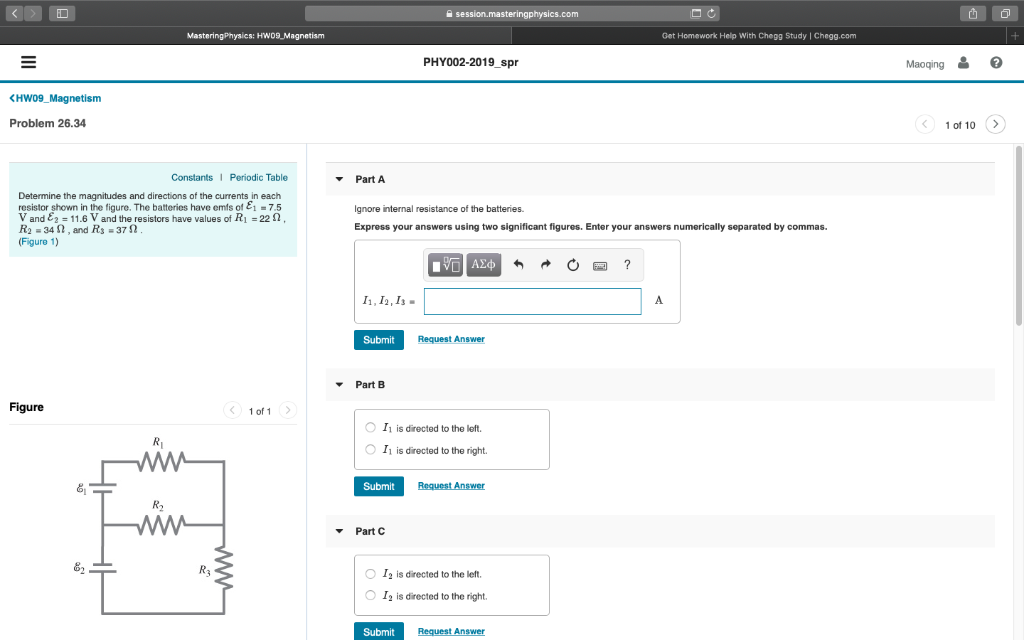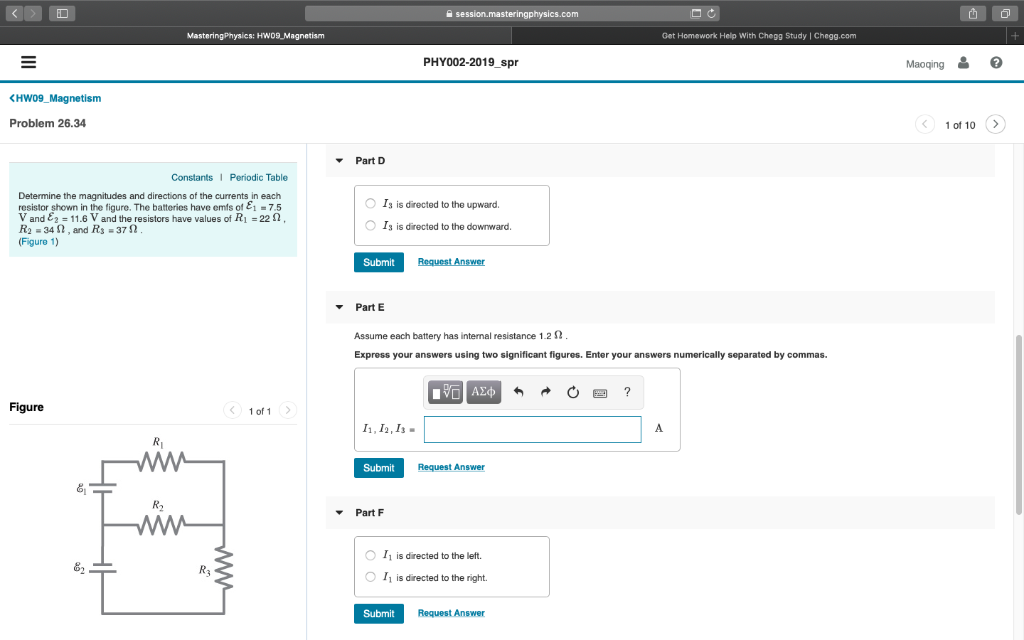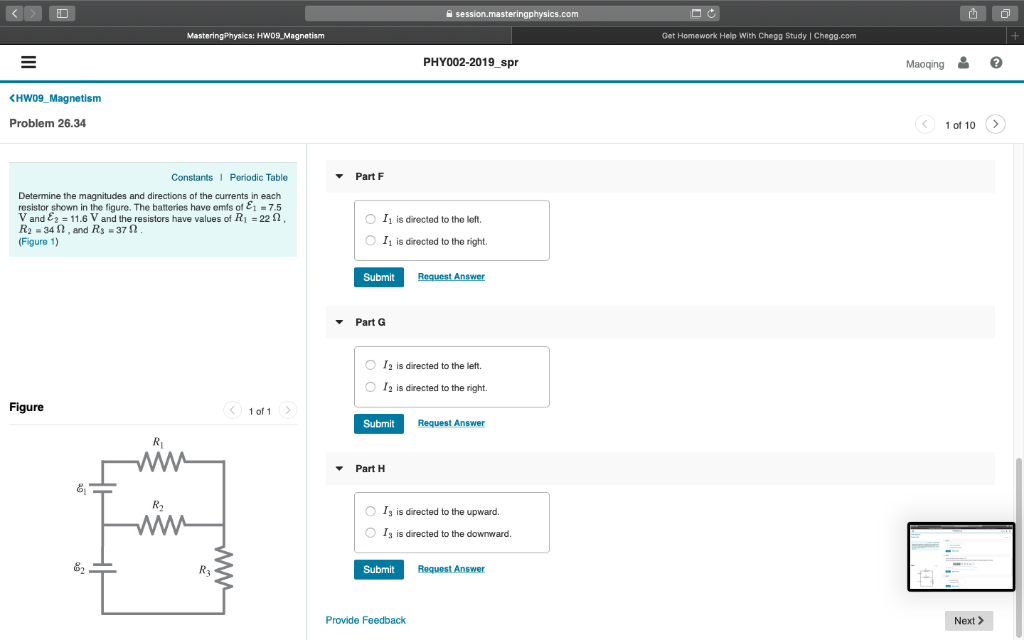Get Homework Help With Chegg Study | Chegg.com PHY002-2019spr Maoging HW09_Magnetism Problem 26.34 1 of 10> Constants I Periodic Table PartA Determine the magnitudes and directions of the currents in each resistor shown in the figure. The batteries have emfs of E1-7.5 V and E2 11.6 V and the resistors have values of R1 22 Ω R2 -34 2, and Rs-372 Figure 1) Ignore internal resistance of the batteries. Express your answers using two significant figures. Enter your answers numerically separated by commas. I1, I, Is Submit Request Answer Part B Figure 1 of 1 O I is directed to the left. O I is directed to the right Submit 81 R2 Part C O I2 is directed to the left I2 is directed to the right. O Submit
Get Homework Help With Chegg Study | Chegg.com PHY002-2019spr Maoqing HW09_Magnetism Problem 26.34 1 of 10> Part D Constants I Periodic Table Determine the magnitudes and directions of the currents in each resistor shown in the figure. The batteries have emfs of E1-7.5 V and E2 11.6 V and the resistors have values of R1 22 Ω R2=34 Ω , and R3=37 Ω Figure 1) O Is is directed to the upward. Is is directed to the downward O Submit Part E Assume each battery has internal resistance 1.2 Ω Express your answers using two significant figures. Enter your answers numerically separated by commas. Figure 1 of 1 I1, I, Is Submit Request Answer 81 R2 Part F O I1 is directed to the left. 11 is directed to the right. O Request Answer Submit
Get Homework Help With Chegg Study | Chegg.com PHY002-2019spr Maoqing Part F Constants I Periodic Table Determine the magnitudes and directions of the currents in each resistor shown in the figure. The batteries have emfs of E1-7.5 V and E2 11.6 V and the resistors have values of R1 22 Ω R2 -34 2, and Rs-372 Figure 1) O I1 is directed to the left. O I1 is directed to the right Submit Part G O I2 is directed to the left. O I2 is directed to the right. Figure 1 of 1 Submit Request Answer Part H 81 R2 O Is is directed to the upward. Is is directed to the downward. O Request Answer Submit Provide Feedback Next >

The current in circuit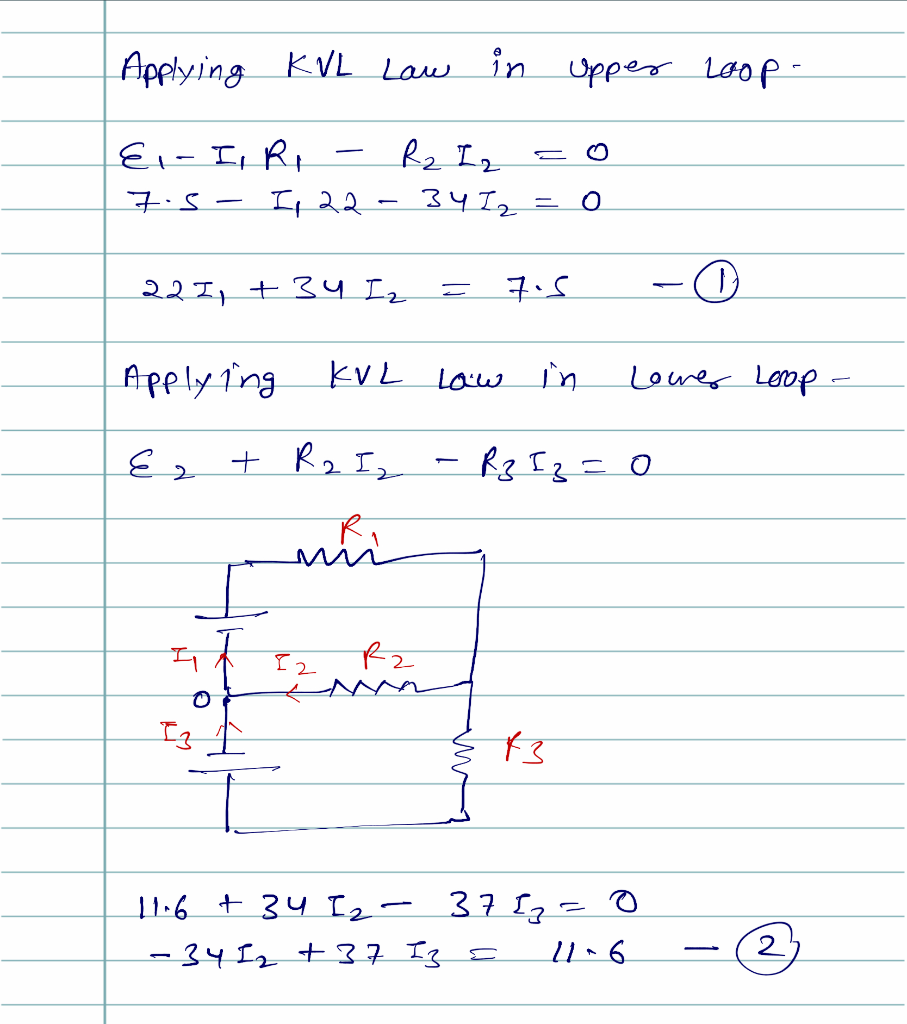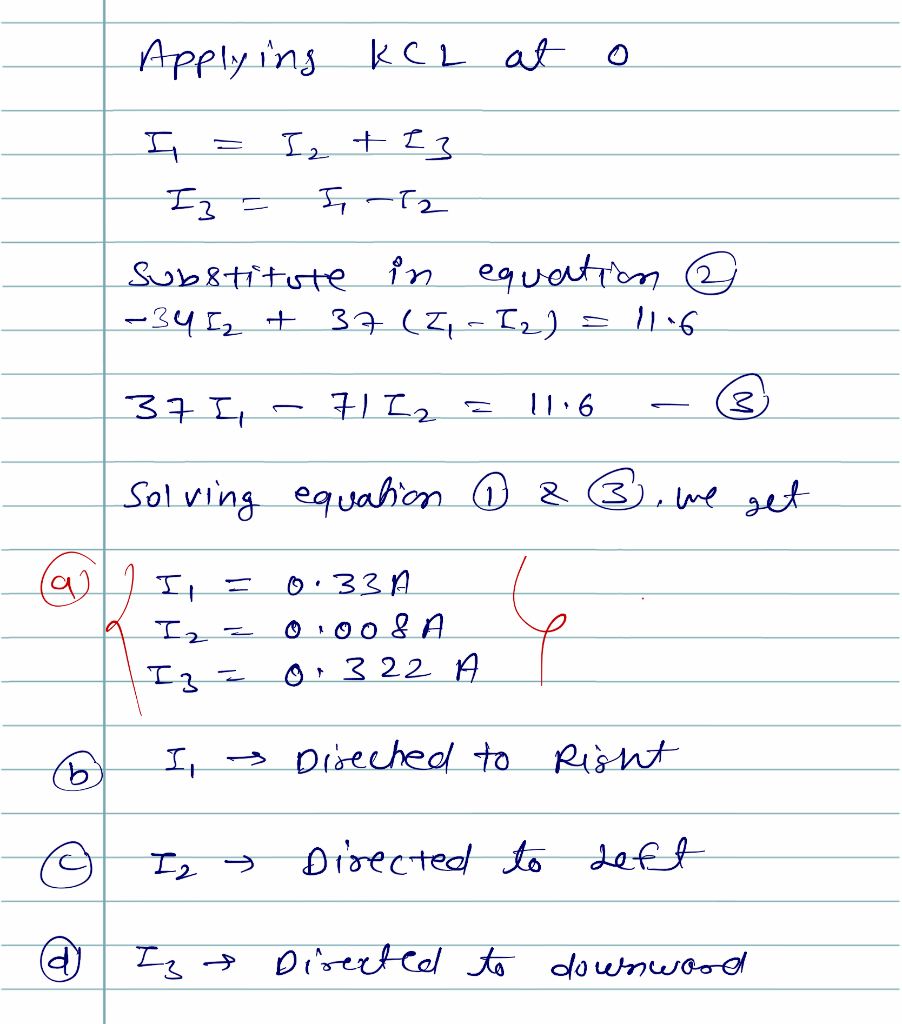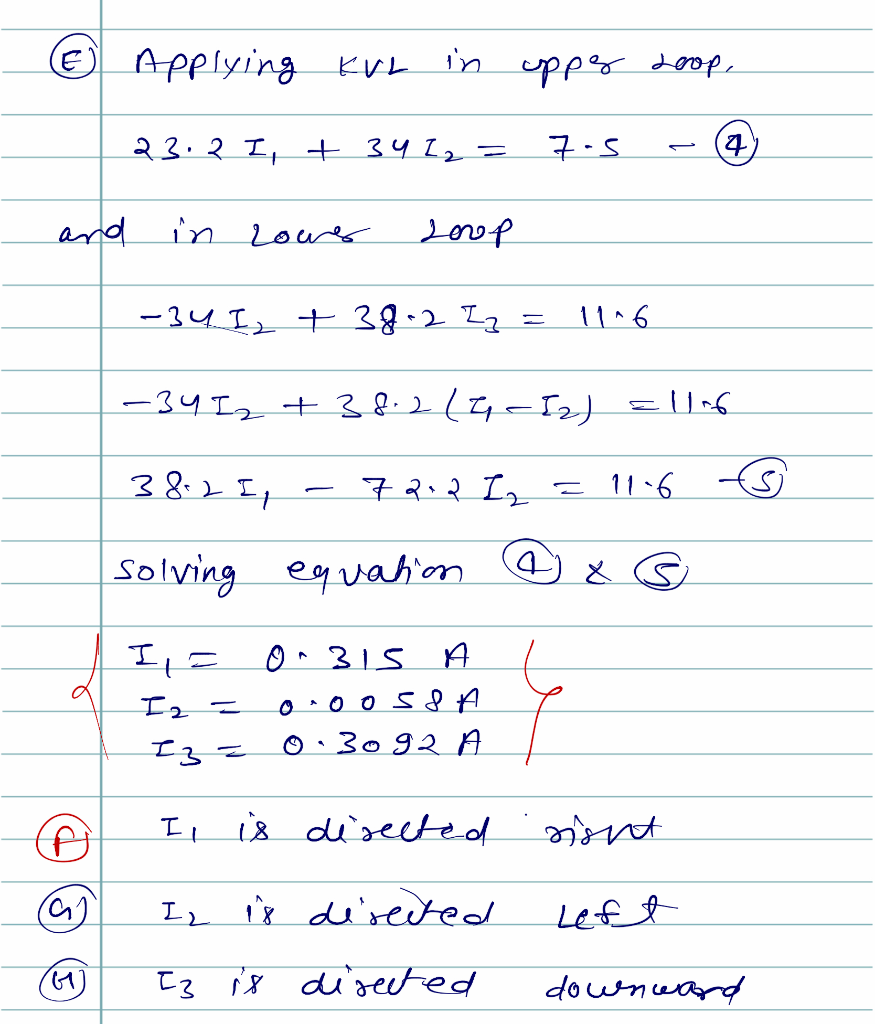##### Add Answer of: Get Homework Help With Chegg Study | Chegg.com PHY002-2019spr Maoging HW09_Magnetism Problem 26.34 1 of 10> Constants I Periodic Table PartA Determine the magnitudes and directions of the currents...
More Homework Help Questions Additional questions in this topic.